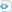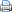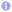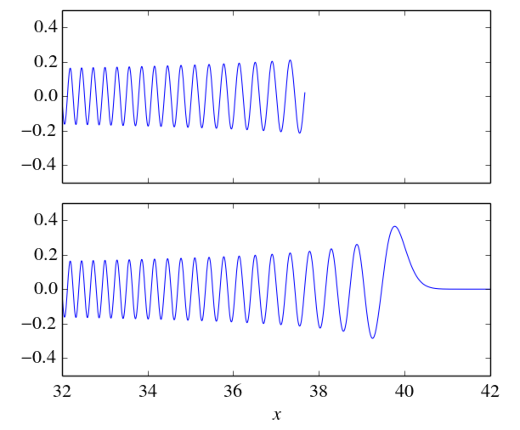numerical methods

# Calculating the Hermite functions

The Hermite functions appear as the solutions of the quantum mechanical harmonic oscillator. But they have applications in many other fields and applications, e.g., pseudospectral methods. The Hermite functions $h_n(x)$ are defined as
\begin{equation}
\label{eq:h}
h_n(x) = \frac{1}{\sqrt{\sqrt{\pi} 2^n n!}} \mathrm{e}^{-x^2/2} H_n(x) \,,
\end{equation} where $H_n(x)$ denotes the $n$th Hermite polynomial defined via the recurrence relation
\begin{equation}
H_{n}(x) = 2xH_{n-1}(x)-2(n-1)H_{n-2}(x)
\end{equation} with the initial values $H_0(x)=1$ and $H_1(x)=2x$. Although the Hermite functions are quite well behaved, they are rather difficult to calculate especially for large $n$ and/or large $x$.

Calculating the Hermite functions via the definition \eqref{eq:h} fails easily due to numerical overflow or underflow that is caused by the rapid growth or decrease of the individual factors $\frac{1}{\sqrt{\sqrt{\pi} 2^n n!}} \mathrm{e}^{-x^2/2}$ and $H_n(x)$. A partial solution to this problem is to introduce the modified Hermite polynomials defined via the recurrence relation
\begin{equation}
\label{eq:h2}
\tilde H_{n}(x) = \sqrt{\frac{2}{n}}x\tilde H_{n-1}(x)-\sqrt{\frac{n-1}{n}}\tilde H_{n-2}(x)
\end{equation} with the initial values $\tilde H_0(x)=1/\sqrt{\pi}$ and $\tilde H_1(x)=\sqrt{2}\,x/\sqrt{\pi}$. With these polynomials the Hermite functions become
\begin{equation}
h_n(x) = \mathrm{e}^{-x^2/2} \tilde H_n(x) \,.
\end{equation} Because the modified Hermite polynomials $\tilde H_{n}(x)$ grow much more slowly than the standard Hermite polynomials we got rid of the normalizing factor $\frac{1}{\sqrt{\sqrt{\pi} 2^n n!}}$.

But this does not solve all problems of underflow and overflow. For $x>38$ the factor $\mathrm{e}^{-x^2/2}$ is smaller than any number that can be represented as a double precision floating point number. A very robust remedy to numerical underflow was sketched in BIT Numerical Mathematics, Vol. 49, pp 281-295. The basic idea is to keep the magnitude of the modified Hermite polynomials on the order of one during their calculation via the recurrence relation \eqref{eq:h2} by introducing a suitable normalizing factor. During the calculation one has to keep track of the sum of the logarithms of these normalizing factors. Then the factor $\mathrm{e}^{-x^2/2}$ has to be modified by this sum when the value of the Hermite function is calculated. A Python implementation of this algorithm is shown below. This algorithm allows to calculate the values of high-order Hermite functions even for quite large arguments.

 Source codefrom numpy import *
from pylab import *

def h(n, x):
if n==0:
return ones_like(x)*pi**(-0.25)*exp(-x**2/2)
if n==1:
return sqrt(2.)*x*pi**(-0.25)*exp(-x**2/2)
h_i_2=ones_like(x)*pi**(-0.25)
h_i_1=sqrt(2.)*x*pi**(-0.25)
sum_log_scale=zeros_like(x)
for i in range(2, n+1):
h_i=sqrt(2./i)*x*h_i_1-sqrt((i-1.)/i)*h_i_2
h_i_2, h_i_1=h_i_1, h_i
log_scale=log(abs(h_i)).round()
scale=exp(-log_scale)
h_i=h_i*scale
h_i_1=h_i_1*scale
h_i_2=h_i_2*scale
sum_log_scale+=log_scale
return h_i*exp(-x**2/2+sum_log_scale)I would like to thank Randolf Beerwerth for drawing my attention to this problem.

Edit 12.06.2020: A typo in eq. \eqref{eq:h2} has been fixed.

## 4 thoughts on “Calculating the Hermite functions”

1. F says:

The code provided is incomplete. “norm” is not defined.

2. Heiko Bauke says:

Well spotted. Thanks. Python code has been fixed.

3. Andre says:

Hi,

thanks for the excellent post.

I notice that there is a problem with the case n=1.

At this line there is an extra exp(-x**2/2)
return sqrt(2.)*x*exp(-x**2/2)*pi**(-0.25)*exp(-x**2/2)

It should be just:
return sqrt(2.)*x*exp(-x**2/2)*pi**(-0.25)

4. Heiko Bauke says:

Thanks for drawing my attention to this typo. I will fix it.